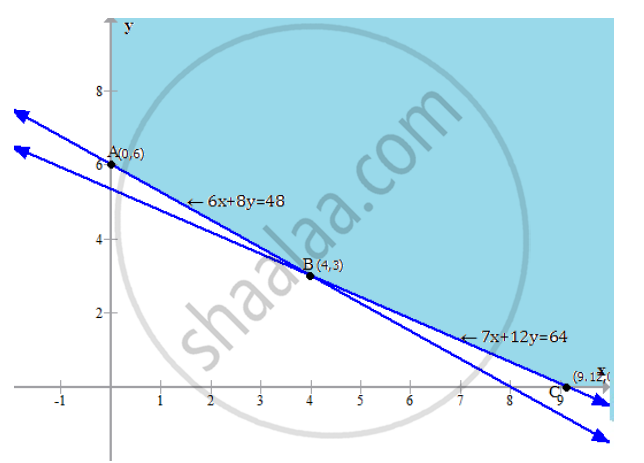HSC Science (Computer Science) 12th Board ExamMaharashtra State Board
Share

Books Shortlist

A Diet of a Sick Person Must Contain at Least 48 Units of Vitamin a and 64 Units of Vitamin B. Two Foods F 1 and F2 Are Available. Food F1 Costs Rs. 6 per Unit and Food F2 Costs Rs. 10 per Unit. One Unit of Food F1 Contains 6 Units of Vitamin a and 7 Units of Vitamin B. - HSC Science (Computer Science) 12th Board Exam - Mathematics and Statistics

ConceptDifferent Types of Linear Programming Problems

Question

A diet of a sick person must contain at least 48 units of vitamin A and 64 units of vitamin B. Two foods F 1 and F2 are available. Food F1 costs Rs. 6 per unit and food F2 costs Rs. 10 per unit. One unit of food F1 contains 6 units of vitamin A and 7 units of vitamin B. One unit of food F2 contains 8 units of vitamin A and 12 units of vitamin B.Find the minimum cost for the diet that consists of mixture of these two foods and also meeting the minimal nutritional requirements.

Solution

Let x and y be two different types of food.
Thus, our objective function is minimise the cost
Z = 6x + 10y, subject to the constraints,
6x + 8y ≥48
7x + 12y ≥ 64
Plotting the above lines in a graph, we have,Thus, the region above ABC is unbounded.
Let us check the value of the function at the corner points A, B and C

 Corner point Value of Z = 6x + 10y (0,6) Z = 0 + 10 x 6 = 60 (4,3) Z = 6 x 4 + 10 x 3 = 54 (64/7,0) Z = 6 x 64/7+ 10 x 0 = 54.85

Minimum of the function is at 4, 3
Minimum cost of the optimum diet is Rs. 54

Is there an error in this question or solution?

APPEARS IN

2014-2015 (March) (with solutions)
Question 3.2.3 | 4.00 marks

Video TutorialsVIEW ALL 

Solution A Diet of a Sick Person Must Contain at Least 48 Units of Vitamin a and 64 Units of Vitamin B. Two Foods F 1 and F2 Are Available. Food F1 Costs Rs. 6 per Unit and Food F2 Costs Rs. 10 per Unit. One Unit of Food F1 Contains 6 Units of Vitamin a and 7 Units of Vitamin B. Concept: Different Types of Linear Programming Problems.
S